# A List Of Epidemics

A list of epidemic events caused by infectious diseases

## Examples

### Basic Examples

Explore the data:

 In:=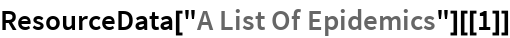Out=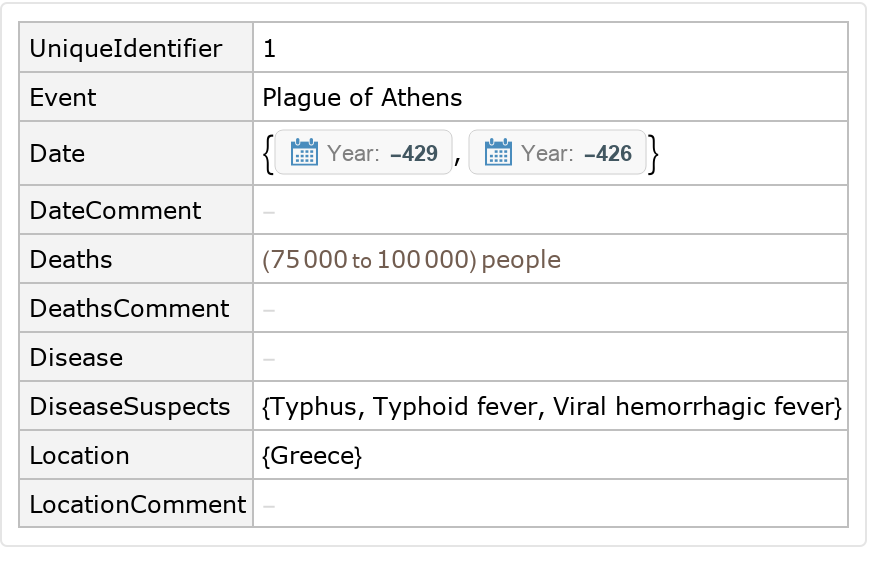### Visualizations

Make a word cloud of infectious diseases by deaths :

 In:=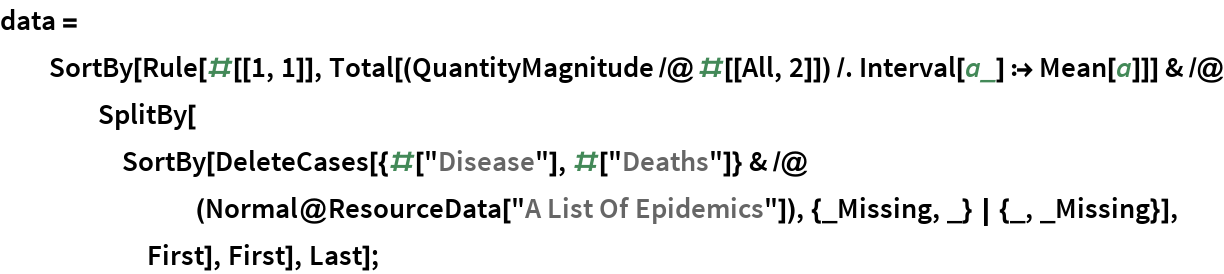In:=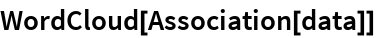Out=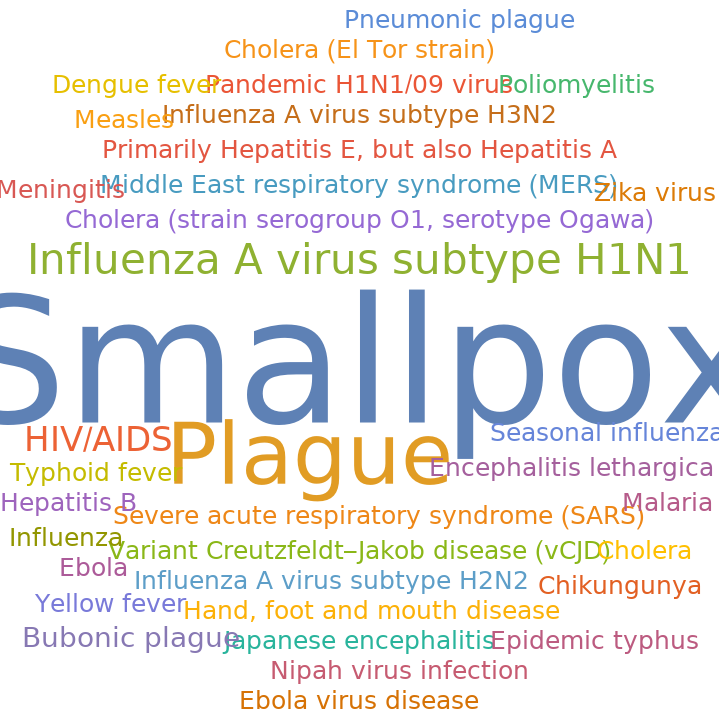Plot a timeline of flu events :

 In:=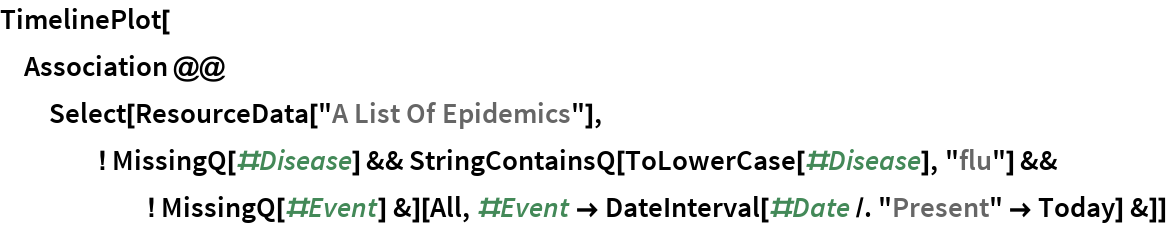Out=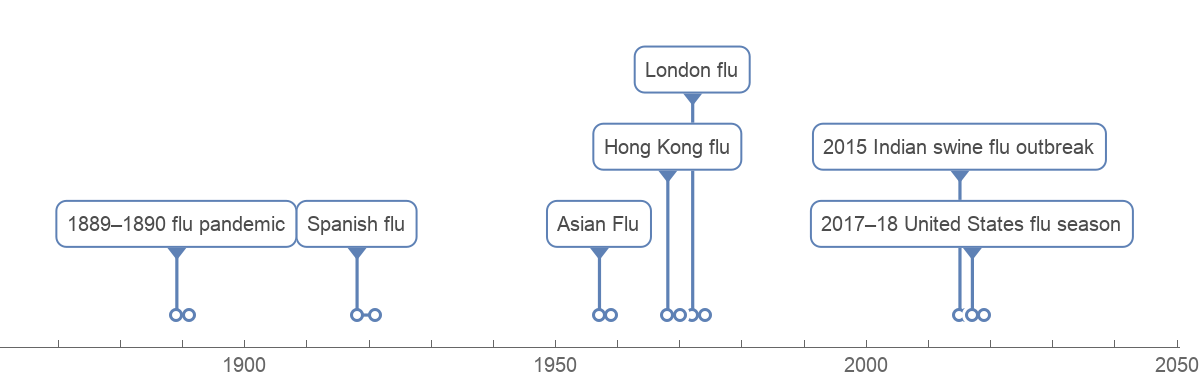Plot a timeline of plague events :

 In:=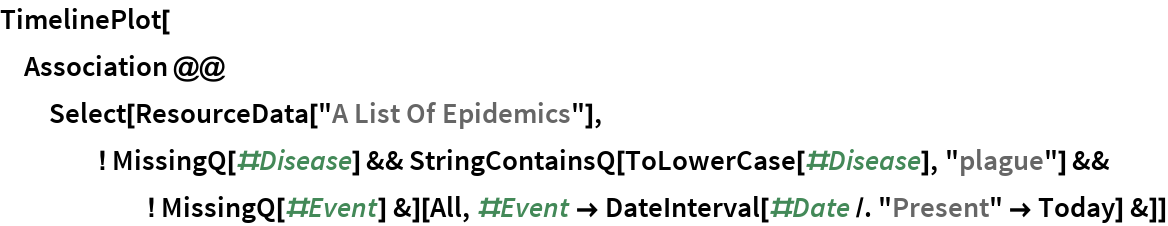Out=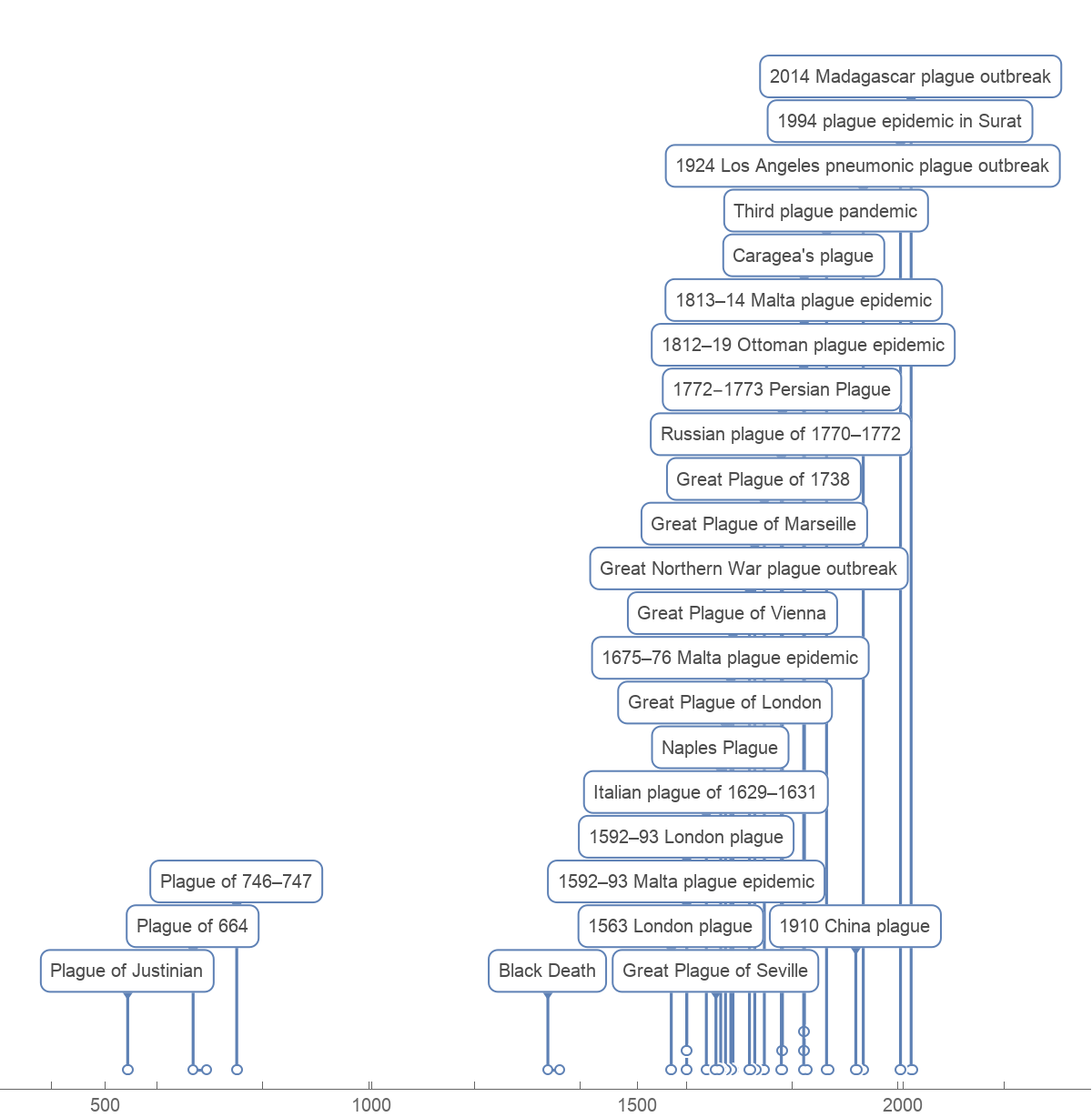Plot a timeline of smallpox events :

 In:=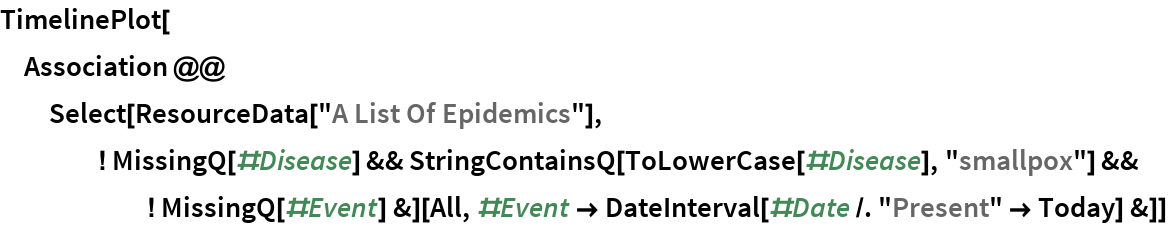Out=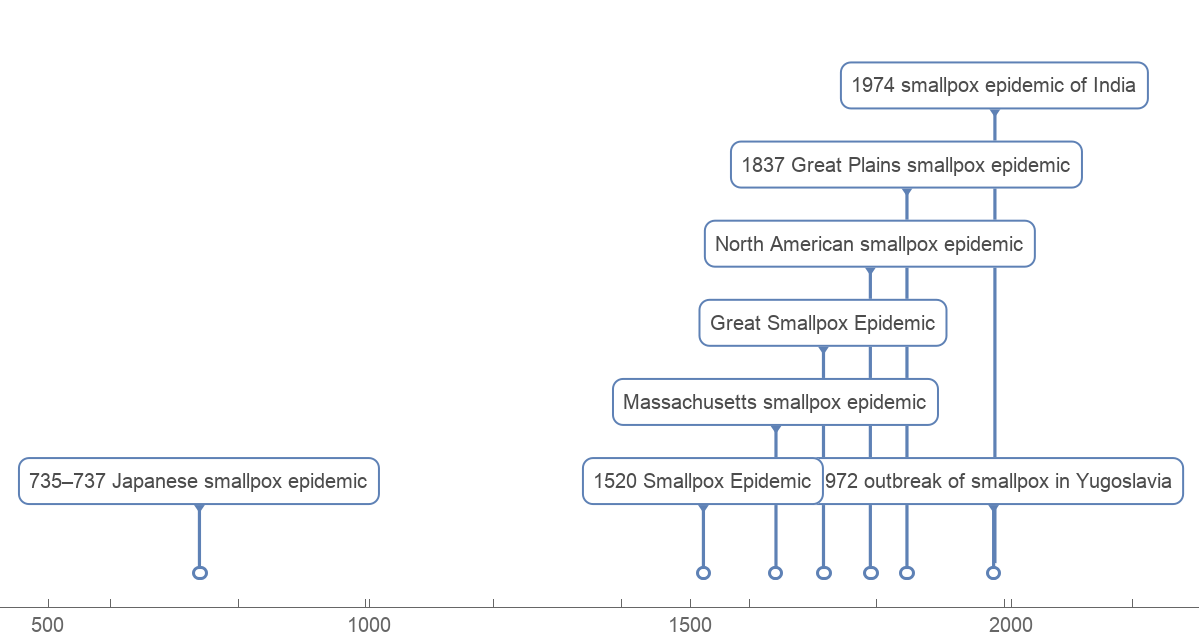Plot a timeline of cholera events :

 In:=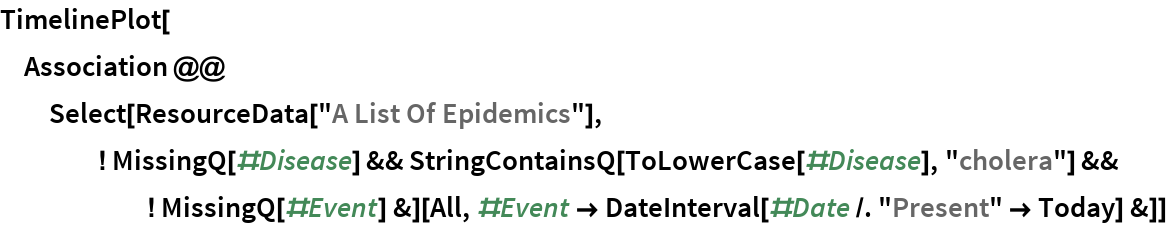Out=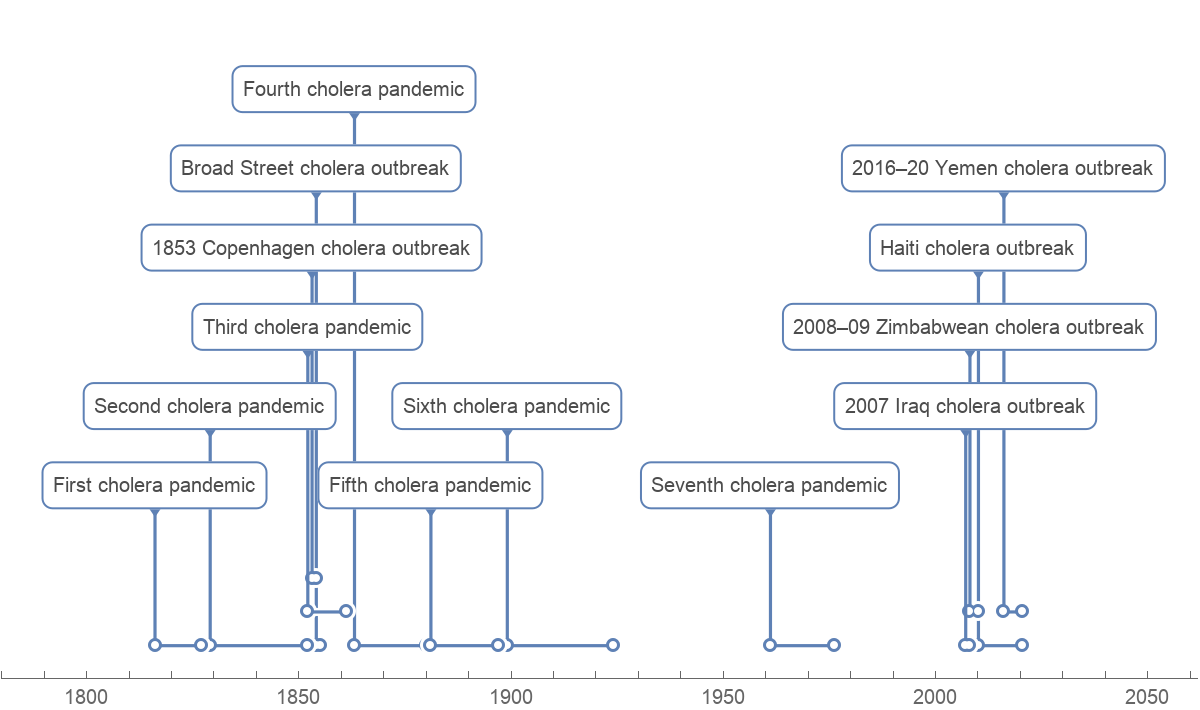Wolfram Research, "A List Of Epidemics" from the Wolfram Data Repository (2020)